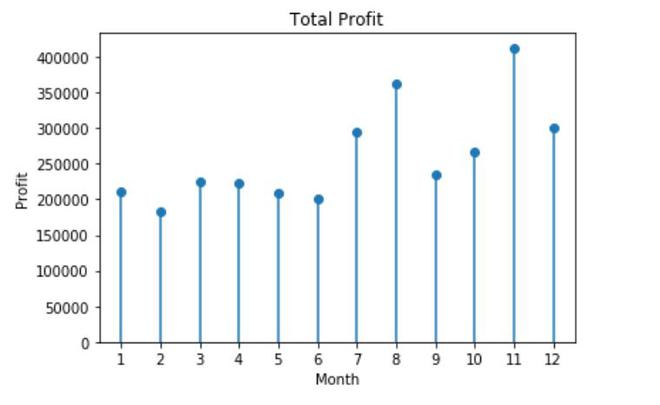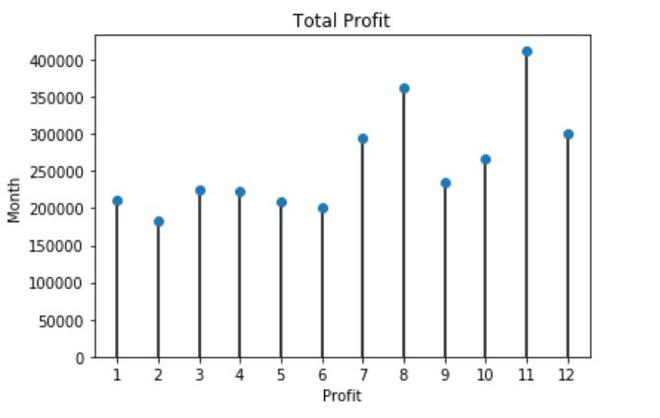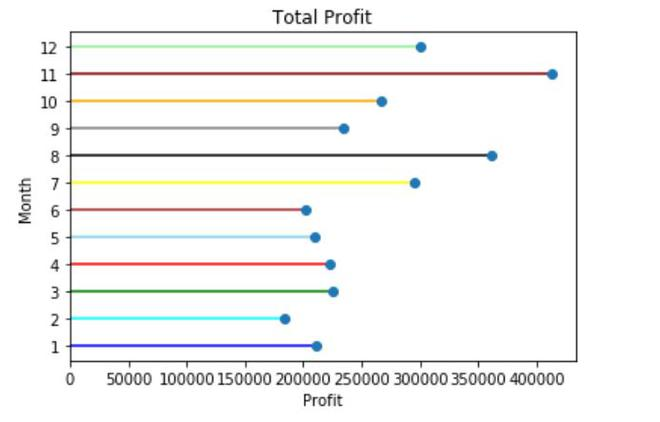Related Articles

# Create lollipop charts with Pandas and Matplotlib

• Difficulty Level : Medium
• Last Updated : 24 Jan, 2021

In this article, we will create Lollipop Charts. They are nothing but a variation of the bar chart in which the thick bar is replaced with just a line and a dot-like “o” (o-shaped) at the end. Lollipop Charts are preferred when there are lots of data to be represented that can form a cluster when represented in the form of bars.

Let’s discuss some examples with different methods to visualize the lollipop chart using pandas.

Example 1:

Using plt.stem function, it creates vertical lines at each x position covered under the graph from the baseline to y and places a marker there. But it is inflexible so you might want to try other methods also.

In this example, we will plot the lollipop chart by collecting the data from a CSV file using pandas.

At first, we will create an empty chart through plt.subplots() and using the stem plot that accepts arguments in the form of (x,y) both can be of array-like values we will plot the values into the chart. The use_line_collection=True improves the performance of the stem plot and plot the stem lines as a LineCollection instead of individual lines. The basefmt=’ ‘ removes the baseline from the stem lines.

The CSV file has column as “month_number” and “total_profit” which has been read from the CSV file and passed into the stem plot as (x,y) and some formatting and details have also been added.

The set_ylim() is set to zero so that the y-axis values start from zero.

Approach:

• Import modules (matplotlib and pandas)
• Open the CSV file and read the data
• Plot it using plt.stem

## Python3

 `# importing modules``from` `pandas ``import` `*``import` `matplotlib.pyplot as plt`` ` `# reading CSV file``d ``=` `read_csv(``"company_sales_data.csv"``)`` ` `# creating an empty chart``fig, axes ``=` `plt.subplots()`` ` `# plotting using plt.stem``axes.stem(d[``'month_number'``], d[``'total_profit'``],``          ``use_line_collection``=``True``, basefmt``=``' '``)`` ` `# starting value of y-axis``axes.set_ylim(``0``)`` ` `# details and formatting of chart``plt.title(``'Total Profit'``)``plt.xlabel(``'Month'``)``plt.ylabel(``'Profit'``)``plt.xticks(d[``'month_number'``])`

Output:Example 2:

In this example, we will create an empty graph/chart using plt.subplots() and then after reading the data from the columns of the CSV file we will draw vertical lines by using vlines() function that takes arguments as (x,ymin,ymax) where ymin is the least value and ymax is the greatest value which is to be plotted.

After drawing the vertical lines we will use the plot() function to draw the markers which will be in the shape of circles and finally the lollipop chart will be formed.

## Python3

 `# import modules``from` `pandas ``import` `*``from` `matplotlib ``import` `pyplot as plt`` ` `# read csv file``d ``=` `read_csv(``"company_sales_data.csv"``)`` ` `# using subplots() to draw vertical lines``fig, axes ``=` `plt.subplots()``axes.vlines(d[``'month_number'``], ymin``=``0``, ymax``=``d[``'total_profit'``])`` ` `# drawing the markers (circle)``axes.plot(d[``'month_number'``], d[``'total_profit'``], ``"o"``)``axes.set_ylim(``0``)`` ` `# formatting and details``plt.xlabel(``'Month'``)``plt.ylabel(``'Profit'``)``plt.title(``'Total Profit'``)``plt.xticks(d[``'month_number'``])`

Output:Example 3:

This example is same as the second method the only difference is in the plotting of the lines, here we will plot the lines horizontally. So, we will create an empty graph/chart using plt.subplots() and then after reading the data from the columns of the CSV file we will draw vertical lines by using hlines() function that takes argument as (y,xmin,xmax) where xmin is the least value and xmax is the greatest value which is to be plotted.

After drawing the vertical lines again we will use the plot() function to draw the markers which will be in the shape of circles and finally the lollipop chart will be formed. Here we will add different colors to each month so that it looks more attractive, the hlines() function as the option colors to set colors to different lines.

## Python3

 `# import modules``from` `pandas ``import` `*``from` `matplotlib ``import` `pyplot as plt`` ` `# read csv file``d ``=` `read_csv(``"company_sales_data.csv"``)`` ` `# using subplots() to draw vertical lines``fig, axes ``=` `plt.subplots()`` ` `# providing list of colors``line_colors ``=` `[``'blue'``, ``'cyan'``, ``'green'``, ``'red'``,``               ``'skyblue'``, ``'brown'``, ``'yellow'``,``               ``'black'``, ``'grey'``, ``'orange'``, ``'maroon'``,``               ``'lightgreen'``]`` ` `axes.hlines(d[``'month_number'``], xmin``=``0``,``            ``xmax``=``d[``'total_profit'``], colors``=``line_colors)`` ` `# drawing the markers (circle)``axes.plot(d[``'total_profit'``], d[``'month_number'``], ``"o"``)``axes.set_xlim(``0``)`` ` `# formatting and details``plt.xlabel(``'Profit'``)``plt.ylabel(``'Month'``)``plt.title(``'Total Profit'``)``plt.yticks(d[``'month_number'``])`

Output:Attention geek! Strengthen your foundations with the Python Programming Foundation Course and learn the basics.

To begin with, your interview preparations Enhance your Data Structures concepts with the Python DS Course. And to begin with your Machine Learning Journey, join the Machine Learning – Basic Level Course

My Personal Notes arrow_drop_up